NEET  >  Close Packed Structures

# Close Packed Structures - Chemistry Class 12 - NEET

## What are Close Packed Structures?

In order to analyze the close packing of constituent particles (ions, molecules or atoms) in a lattice, we get into the assumption that constituent particles (ions, molecules or atoms) are hard spheres of identical shape.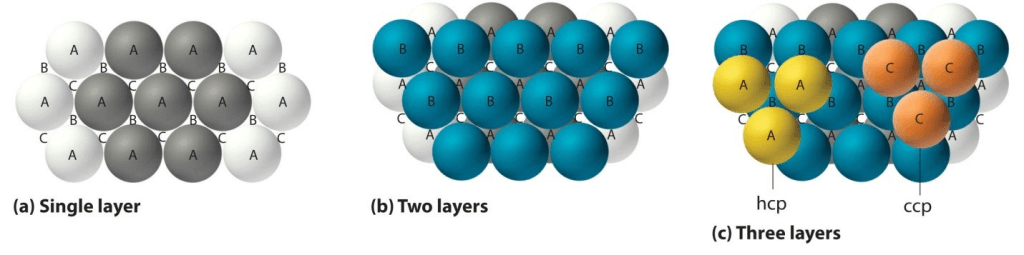Image 1: Different Types of Layers form closed packed structures

What is Close Packing?
The packing of constituent particles inside lattice in such a way that they occupy maximum available space in the lattice is known as Close Packing.
Close Packing is done in three ways, namely:
(a) One Dimensional Close Packing
(b) Two Dimensional Close Packing
(c) Three Dimensional Close Packing

(a) One Dimensional Close Packing
In one dimension close packing, the spheres are arranged in a row touching each other. In one-dimensional close packing, each sphere is in direct contact with two of its neighbor spheres. The number of nearest spheres to a particle in a lattice is called Coordination Number. Since there are two spheres in direct contact with the one sphere, the coordination number of one-dimensional close packing is 2.

(b) Two Dimensional Close Packing
Two-dimensional close packing is done by stacking rows of hard spheres one above the other. This can be done in two ways:
•  AAA type
•  ABA type

AAA Type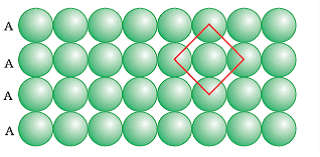Image 2: AA type packing

The packing in which one sphere touches two spheres placed in two different rows one above and one below is called AAA type close packing. The coordination number of AAA type two-dimensional close packing is 4. The AAA type is formed by placing one-dimensional row directly one above the other in both horizontal and vertical directions. It is also called two-dimensional square close packing as the rows of spheres when arranged in vertical and horizontal alignments form a square.

AB Type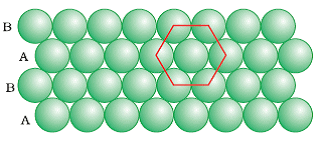Image 3: AB Type Packing

The packing in which the spheres in the second row are located in the depressions of the first row. The ABA type close packing is formed by placing one-dimensional row let’s say B type over the A type close packing and this series continues to form a two dimensional hexagonal. The coordination number of ABA Type packing is 6 as each sphere is in direct contact with 6 other spheres.
In ABA type close-packing we find triangular empty spaces called voids. These are of two types:
•  Apex of triangle pointing upwards
•  Apex of triangle pointing downwards

(c) Three Dimensional Close Packing

The formation of real lattices and structures take place through three-dimensional close packing. They are formed by stacking two-dimensional layers of spheres one above the other. This can be done by two ways:

• Three-dimensional close packing from two dimensional square close packed layers
• Three-dimensional close packing from two dimensional hexagonal close packed layers

Three-dimensional close packing from two-dimensional close packed layers
Formation of three-dimensional close packing can be done by placing the second square closed packing exactly above the first one. In this close packing, the spheres are aligned properly in horizontally and vertically. Similarly, by placing more layers one above the other, we can obtain a simple cubic lattice. The unit cell of the simple cubic lattice is called the primitive cubic unit cell.
Three-dimensional close packing from two dimensional hexagonal close-packed layers
Three-dimensional close packing can be formed with the help of two-dimensional hexagonal packed layers in two ways:
•  Stacking the second layer over the first layer
•  Stacking the third layer over the second layer

Stacking the second layer over the first layer
Suppose we take two hexagonal close packed layer ‘A’ and place it over the second layer B ( as both layers have different alignment of spheres) such that spheres of the second layer are placed in the depressions of the first layer. We observe that a tetrahedral void is formed when a sphere of the second layer is right above the void (empty space) of the first layer. Adding further we notice octahedral voids at the points where the triangular voids of the second layer are placed right triangular voids of the first one in such a way that triangular space doesn't overlap. Octahedral voids are bordered by six spheres.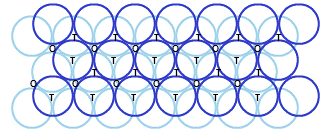Image 4: Types of voids in three-dimensional closed packing

If there are ‘N’ closed spheres, then:
•  Number of Octahedral Voids equals to “N”
•  Number of Tetrahedral Voids equals to “ 2N”
Stacking the third layer over the second layer
There are two possible ways of placing the third layer over the second layer:

(1) By Covering Tetrahedral Voids
(2) By Covering Octahedral Voids

(1) Covering Tetrahedral Voids
In this kind of three-dimensional packing, the spheres of the third layer are aligned right above the spheres of the first layer. If we name the first layer as A and second layer as B, then the pattern will be ABAB… so far and so forth. The structure formed is also called hexagonal close-packed structure also known as HCP.

(2) Covering Octahedral Voids
In this kind of packing the third layer, spheres are not placed with either of the second layer or first layer. If we name the first layer as A, second as B and then the third layer will be C (as it is now a different layer) then the pattern will be ABCABC… The structure formed is also called cubic closed packed (ccp) or face-centred packed cubic structure (fcc). For Example metals like copper and iron crystallize in the structure.
The coordination number in both cases will be 12 as each sphere in the structure is in direct contact with 12 other spheres. The packing is highly efficient and around 74% of the crystal is completely occupied.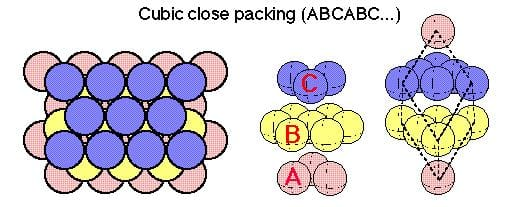ABC Type of Close Packing

The formula of a Compound and Number of Voids Filled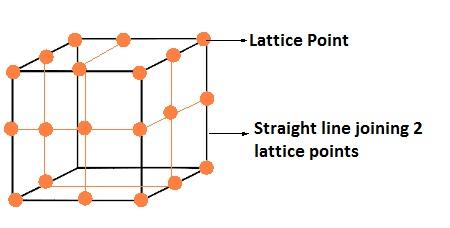• The number of octahedral voids present in a lattice = The number of close-packed particles.

• The number of tetrahedral voids produced is twice this number.

• In ionic solids, the bigger ions i.e. anions forms close-packed structure and the smaller ions i.e. cations occupy the voids.

• If the latter ion is small then tetrahedral voids are occupied, if bigger, then octahedral voids are occupied.

• In a compound, the fraction of octahedral or tetrahedral voids that are occupied depends upon the chemical formula of the compound.

• Close packed structures have both tetrahedral and octahedral voids.

Calculations Involving Unit Cells Dimensions
With the help of geometry and attributes of unit cells, we can easily evaluate the volume of the unit cell. With the volume and mass of atoms, we can also evaluate the density of the unit cell. A crystal lattice is represented in terms of unit cells; we can determine the density of crystal lattices by evaluating the density of unit cells.
Volume Density
Formula volume density of metal:
ρv = mass per unit cell/Volume per unit cell

The density of Unit Cell
A unit cell is a three-dimensional structure occupying one, two or more atoms. With the help of the dimensions of unit cells, we can evaluate the density of the unit cell. To do so let’s consider a unit cell of edge length ‘a’, therefore the volume of the cell will be ‘a3’. Also, density is defined as the ratio of the mass of the unit cell and volume of the unit cell.

The density of Unit Cell = mass of unit cell/Volume of unit cell......(1)

Mass of unit cell varies with number of atoms “n” and mass of a single atom “m”. Mathematically mass of unit cell is the product of number of atoms “n” and mass of one atom “m” i.e.
Mass of Unit Cell = n × m
Also from quantitative aspect of atoms, mass of one atom can be written in terms of Avogadro Number ( NA ) and molar mass of atom ( M), that is,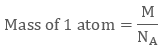Volume of Unit Cell = a3
Placing the required values in equation 1 we get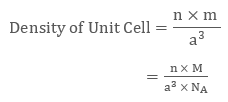Therefore if we know molar mass of atom “M”, number of atoms “n”, the edge length of unit cell “a” we can evaluate the density of unit cell.

Example 1
The density of a face-centred unit cell is 6.23 g cm-3. Given the atomic mass of a single atom is 60, evaluate the edge length of the unit cell. (Take value of NA = 6.022 × 1023)
As the unit cell is a face-centred unit cell, a number of atoms will be  4. We have
n = 4 , M = 60 , NA = 6.022 × 1023 and d = 6.23 g cm-3
We know that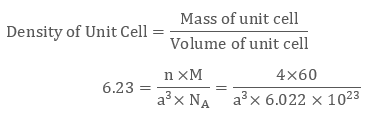After evaluating we get the value of edge length of the cube to be 4 × 10-8 cm.

Example 2
KBr or potassium bromide has density 2.75 g cm-3. The edge length of its unit cell is 654 pm. Prove that KBr depicts face-centred cubic structure.
We have edge length of unit cell = 654 pm = 6.54 × 10-8 cm
Therefore volume of the cell = (6.54 ×10-8)3 cm3
Molar Mass of Potassium Bromide ( KBr) is 119 g/mol
Density of KBr = 2.75 g cm-3
We know that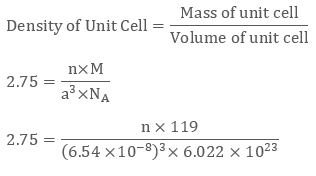Evaluating we get value of n to be 3.09 ≈ 4
Since a number of atoms is 4 we can clearly say that KBr is a face-centred cubic structure.

The document Close Packed Structures | Chemistry Class 12 - NEET is a part of the NEET Course Chemistry Class 12.
All you need of NEET at this link: NEET

## Chemistry Class 12

150 videos|373 docs|213 tests

## FAQs on Close Packed Structures - Chemistry Class 12 - NEET

 1. What are close packed structures?Ans. Close packed structures refer to the arrangement of atoms or particles in a way that maximizes the packing efficiency. In these structures, the atoms are arranged in a closely packed manner, resulting in minimal empty space between them.
 2. What are the types of close packed structures?Ans. There are two types of close packed structures: hexagonal close-packed (HCP) and cubic close-packed (CCP). In HCP, the atoms are arranged in a hexagonal pattern, while in CCP, the atoms are arranged in a cubic pattern.
 3. How do close packed structures determine the physical properties of materials?Ans. Close packed structures play a crucial role in determining the physical properties of materials. The packing efficiency affects properties such as density, strength, and electrical conductivity. For example, close packed structures generally have higher densities due to the efficient arrangement of atoms.
 4. How are close packed structures formed?Ans. Close packed structures are formed through the stacking of layers of atoms. In HCP, the layers are stacked in an ABAB pattern, while in CCP, the layers are stacked in an ABCABC pattern. This stacking arrangement allows for the close packing of atoms.
 5. What are some examples of materials with close packed structures?Ans. Some examples of materials with close packed structures include metals such as copper, silver, and gold. These metals exhibit either HCP or CCP structures, which contribute to their high ductility, conductivity, and luster.

## Chemistry Class 12

150 videos|373 docs|213 testsExplore Courses for NEET exam### How to Prepare for NEET

Read our guide to prepare for NEET which is created by Toppers & the best Teachers
Signup to see your scores go up within 7 days! Learn & Practice with 1000+ FREE Notes, Videos & Tests.
10M+ students study on EduRev
Track your progress, build streaks, highlight & save important lessons and more!
Related Searches

,

,

,

,

,

,

,

,

,

,

,

,

,

,

,

,

,

,

,

,

,

;